Shortcuts

# Theil’s U¶

## Module Interface¶

class torchmetrics.TheilsU(num_classes, nan_strategy='replace', nan_replace_value=0.0, **kwargs)[source]

Compute Theil’s U statistic (Uncertainty Coefficient) measuring the association between two categorical (nominal) data series.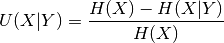where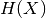is entropy of variable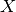while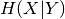is the conditional entropy ofgiven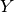.

Theils’s U is an asymmetric coefficient, i.e.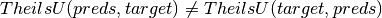.

The output values lies in [0, 1]. 0 means y has no information about x while value 1 means y has complete information about x.

Parameters
Returns

Tensor

Return type

Theil’s U Statistic

Example

>>> from torchmetrics import TheilsU
>>> _ = torch.manual_seed(42)
>>> preds = torch.randint(10, (10,))
>>> target = torch.randint(10, (10,))
>>> TheilsU(num_classes=10)(preds, target)
tensor(0.8530)


Initializes internal Module state, shared by both nn.Module and ScriptModule.

compute()[source]

Computer Theil’s U statistic.

Return type

Tensor

update(preds, target)[source]

Update state with predictions and targets.

Parameters
• preds (Tensor) – 1D or 2D tensor of categorical (nominal) data

• shape (- _sphinx_paramlinks_torchmetrics.TheilsU.update.2D) – (batch_size,)

• shape – (batch_size, num_classes)

target: 1D or 2D tensor of categorical (nominal) data
• 1D shape: (batch_size,)

• 2D shape: (batch_size, num_classes)

Return type

None

## Functional Interface¶

torchmetrics.functional.theils_u(preds, target, nan_strategy='replace', nan_replace_value=0.0)[source]

Compute Theil’s U statistic (Uncertainty Coefficient) measuring the association between two categorical (nominal) data series.whereis entropy of variablewhileis the conditional entropy ofgiven.

Theils’s U is an asymmetric coefficient, i.e..

The output values lies in [0, 1]. 0 means y has no information about x while value 1 means y has complete information about x.

Parameters
Returns

Tensor

Return type

Theil’s U Statistic

Example

>>> from torchmetrics.functional import theils_u
>>> _ = torch.manual_seed(42)
>>> preds = torch.randint(10, (10,))
>>> target = torch.randint(10, (10,))
>>> theils_u(preds, target)
tensor(0.8530)


### theils_u_matrix¶

torchmetrics.functional.nominal.theils_u_matrix(matrix, nan_strategy='replace', nan_replace_value=0.0)[source]

Compute Theil’s U statistic between a set of multiple variables.

This can serve as a convenient tool to compute Theil’s U statistic for analyses of correlation between categorical variables in your dataset.

Parameters
Return type

Tensor

Returns

Theil’s U statistic for a dataset of categorical variables

Example

>>> from torchmetrics.functional.nominal import theils_u_matrix
>>> _ = torch.manual_seed(42)
>>> matrix = torch.randint(0, 4, (200, 5))
>>> theils_u_matrix(matrix)
tensor([[1.0000, 0.0202, 0.0142, 0.0196, 0.0353],
[0.0202, 1.0000, 0.0070, 0.0136, 0.0065],
[0.0143, 0.0070, 1.0000, 0.0125, 0.0206],
[0.0198, 0.0137, 0.0125, 1.0000, 0.0312],
[0.0352, 0.0065, 0.0204, 0.0308, 1.0000]])


© Copyright Copyright (c) 2020-2023, Lightning-AI et al... Revision 6853c553.

Built with Sphinx using a theme provided by Read the Docs.
Versions
latest
stable
v0.11.2
v0.11.1
v0.11.0
v0.10.3
v0.10.2
v0.10.1
v0.10.0
v0.9.3
v0.9.2
v0.9.1
v0.9.0
v0.8.2
v0.8.1
v0.8.0
v0.7.3
v0.7.2
v0.7.1
v0.7.0
v0.6.2
v0.6.1
v0.6.0
v0.5.1
v0.5.0
v0.4.1
v0.4.0
v0.3.2
v0.3.1
v0.3.0
v0.2.0
v0.1.0